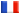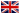# Séminaire de Cryptographie

## Razvan Basbulescu### A heuristic quasi-polynomial algorithm for discrete logarithm

in finite fields of small characteristic The difficulty of discrete logarithm computations in fields GF(q^k) depends on the relative sizes of k and q. Until recently all the cases had a sub-exponential complexity of type L(1/3), similar to the complexity of factoring. If n is the bit-size of q^k, then L(1/3) can be approximated by 2^(n^(1/3)). In 2013, Joux designed a new algorithm for constant characteristic of complexity L(1/4+o(1)), approximatively 2^(n^(1/4)). Inspired by Joux' algorithm, we propose a heuristic algorithm that provides a quasi-polynomial complexity when q is of size O(poly(k)). By quasi-polynomial, we mean a runtime of n^O(log n).

Hence, small characteristic pairings have an asymptotic complexity which is inapropiate for cryptography. In addition, in practice we expect the algorithm to be much faster in the case GF(q^2k), when q and k are roughly equal. The small characteristic pairings which were previously evaluated to 128 bits of security correspond to this case, and were reevaluated to a much lower security. It allows to conclude that small characteristic pairings must be avoided in cryptography.